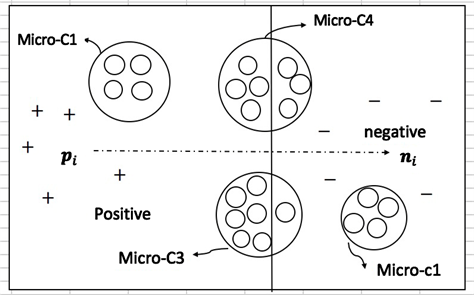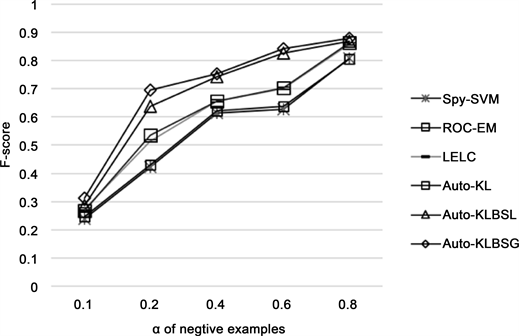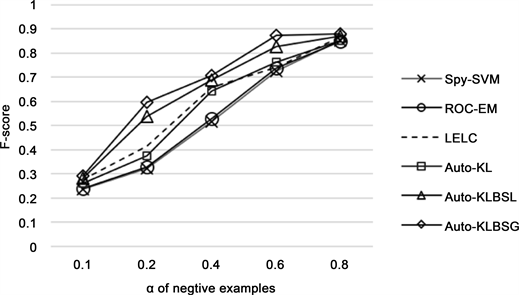# 数据分布估计下基于相似度的PU文本分类方法PU Text Classification Method Based on Similarity under Data Distribution Estimation

• 全文下载: PDF(1035KB)    PP.788-797   DOI: 10.12677/CSA.2018.85088
• 下载量: 432  浏览量: 1,550   国家自然科学基金支持

In actual applications, due to various reasons, it is usually impossible to obtain the marked negative data, which causes the traditional classification algorithm to fail. Based on positive data and unlabeled data learning, it is called the PU classification problem. The key of the PU problem lies in the extraction of negative data and the construction of effective classifiers. The algorithm proposed in this paper firstly evaluates the data distribution in the sample, adopts the integration mechanism to extract positive and negative example data from the unlabeled sample with reasonable proportion, and then uses similarity to extract the representative positive micro-clusters and negative micro-clusters. After sufficient samples of positive and negative samples were obtained, the PU problem was converted to a binary classification problem. Numerical experiments showed the effectiveness of the method.

1. 引言

Hu等人  提出一种可以评估未标注数据中数据分布的算法Auto-KL，其核心思想是先利用当前分类任务所提供的数据样本生成不同数据分布比例的模拟数据，然后根据模拟数据训练数据分布评估分类模型，最后根据当前数据进行数据分布估计。从而保证从未标注数据中抽取的足够多的有用信息，同时也尽量避免抽取到错误信息。但是剩余的未标注数据中仍有大量数据未被利用，从而使训练出来的分类器泛化能力不强。

2. 研究现状

1) 忽略未标注数据集，例如Tax等人  提出的SVDD方法，Mabevtz等人  提出了一种单类SVM  方法用于文本分类中。这类方法由于完全忽略了未标注数据集，从而丢失了未标注数据集中隐藏的有用信息，例如未标注数据集中存在可靠的反例样本时，忽略这类信息极易出现过拟合的现象。

2) 利用未标注数据集中有效信息增强训练模型。针对第1类方法的不足，显而易见的方法是考虑将无标签数据加入到训练集中，利用已有的正例样本和加入无标签数据中的知识可以训练获得到更有效的分类器。考虑从无标注数据中提取反例数据，结合已有的正例样本可以训练一个标准的二元分类器。Yu等人  提出了PEBL算法来解决PU分类问题，它首先利用1-DNF  技术来识别未标注数据中的反例数据，然后利用SVM算法训练分类模型。Liu等人  提出S-EM算法，利用Spy  技术识别未标注数据中的可信度较高的反例数据，然后使用EM算法来进行训练模型。Li等人  将传统的PU问题应用到流式数据环境下，提出了一种基于聚类的PU学习算法。Xiao等人  提出了一种基于相似度的PU学习算法，首先利用正例样本提取未标注样本数据集中可信度较高的反例样本，然后基于正例样本与提取的反例样本，计算剩余未标注样本分属正例与反例的概率，基于以上数据建立带有概率权重的SVM分类器。Ren等人  提出了一种基于相似度PU学习算法应用于虚假评论识别领域。但是由于未标注样本正反例样本分布未知，最终分类器效果也会受到抽取反例参数的影响。

3. 数据分布估计下基于相似度的PU文本分方法

3.1. 问题定义及符号标注

3.2. 抽取可信正反例

3.3. 计算代表性正负例原型

1) 输入：P和RN

2) 输出： ${p}_{k}$${n}_{k}$$k=1,\cdots ,10$

3) 将RN聚类成10个子类： ${\text{RN}}_{1},{\text{RN}}_{2},\cdots ,{\text{RN}}_{10}$

4) FOR $k=1,\cdots ,10$ DO

5) ${p}_{k}=\alpha \frac{1}{|\text{P}+\text{RP}|}{\sum }_{e\in p}\frac{e}{‖e‖}-\beta \frac{1}{|{\text{RN}}_{k}|}{\sum }_{e\in R{N}_{k}}\frac{e}{‖e‖}$ ;

6) ${n}_{k}=\alpha \frac{1}{|{\text{RN}}_{k}|}{\sum }_{e\in p}\frac{e}{‖e‖}-\beta \frac{1}{|\text{P}|}$ ;

7) END FOR

3.4. 确定间谍样例的类别标签

3.4.1. 样例局部相似性

1) ${\text{LP}}_{i}=\varnothing ,{\text{LN}}_{i}=\varnothing ,\text{pos_vote}=0,\text{neg_vote}=0$

2) FOR每个样例 $e\in {\text{US}}_{i}$ DO

3) IF ${\mathrm{max}}_{i=1}^{10}sim\left(e,{p}_{i}\right)>{\mathrm{max}}_{i=1}^{10}sim\left(e,{n}_{i}\right)$

4) THEN pos_vote++;

5) ELSE neg_vote++;

6) END IF

7) END FOR

8) IF pos_vote > neg_vote

9) THE ${\text{LP}}_{i}={\text{LP}}_{i}\cup {\text{US}}_{i}$

10) ELSE ${\text{LN}}_{i}={\text{LN}}_{i}\cup {\text{US}}_{i}$

11) END IF

$sim\left(x,y\right)=x\cdot y/‖x‖\cdot ‖y‖.$ (1)

3.4.2. 样例全局相似性Figure 1. Local similarity of the sample

1) ${\text{LP}}_{i}=\varnothing ,{\text{LN}}_{i}=\varnothing$

2) FOR每个样例 $e\in U{S}_{i}$ DO

3) IF proba_positive(e) > proba_negative(e)

4) THE ${\text{LP}}_{i}={\text{LP}}_{i}\cup \left\{e\right\}$

5) ELSE ${\text{LN}}_{i}={\text{US}}_{i}\cup \left\{e\right\}$

6) END IF

7) END FOR

$Proba\text{_}positive\left(e\right)={\sum }_{i=1}^{10}sim\left(e,{p}_{i}\right)/{\sum }_{i=1}^{10}sim\left(e,{p}_{i}\right)+sim\left(e,{n}_{i}\right);$ (2)

$Proba\text{_}negative\left(e\right)={\sum }_{i=1}^{10}sim\left(e,{n}_{i}\right)/{\sum }_{i=1}^{10}sim\left(e,{p}_{i}\right)+sim\left(e,{n}_{i}\right);$ (3)

3.5. 建立最终的分类器

1) $\text{P}=\text{P}\cup \text{LP}$

2) $\text{N}=\text{RN}\cup \text{LN}$

3) 使用SimpleMKL在P+N，训练最终分类器FSimpleMKL

4. 数据分布估计下基于相似度的PU文本分类方法

4.1. 实验与分析

4.1.1. 实验设置

4.1.2. 特征提取

4.1.3. 实验结果与分析Table 2. 6 sets of sub-datasets on each algorithm F-scoreFigure 2. Classification performance comparison on 20-NewsgroupFigure 3. Classification performance comparison on Reuters corpus

5. 数据分布估计下基于相似度的PU文本分类方法

  Liu, B., Lee, W.S., Yu, P.S., et al. (2002) Partially Supervised Classification of Text Documents. Nineteenth Internation-al Conference on Machine Learning, Morgan Kaufmann Publishers Inc., Sydney, July 2002, 387-394.  Tax, D.M.J. (1999) Data Domain Description Using Support Vectors. European Symposium on Artificial Neural Networks’99, Brugge, 21-23 April 1999, 251-256.  Manevitz, L.M. and Yousef, M. (2002) One-Class Svms for Document Clas-sification. Journal of Machine Learning Research, 2, 139-154.  Yu, H., Han, J. and Chang, C.C. (2002) PEBL: Positive Example Based Learning for Web Page Classification Using SVM. Eighth ACM SIGKDD International Con-ference on Knowledge Discovery and Data Mining, Beijing, 12-16 August 2012, 239-248.  Li, X.L., Yu, P.S., Liu, B., et al. (2009) Positive Unlabeled Learning for Data Stream Classification. Siam International Conference on Data Mining, SDM 2009, Sparks, Nevada, 30 April-2 May 2009, 257-268.  Xiao, Y., Liu, B., Yin, J., et al. (2011) Simi-larity-Based Approach for Positive and Unlabeled Learning. IJCAI 2011, Proceedings of the, International Joint Confer-ence on Artificial Intelligence, Barcelona, July 2011, 1577-1582.  Ren, Y., Ji, D. and Zhang, H. (2014) Positive Un-labeled Learning for Deceptive Reviews Detection. Proceedings of the 2014 Conference on Empirical Methods in Natu-ral Language Processing, EMNLP, Doha, 25-29 October 2014, 488-498. https://doi.org/10.3115/v1/D14-1055  Li, X. and Liu, B. (2003) Learning to Classify Texts Using Positive and Unlabeled Data. International Joint Conference on Artificial Intelligence, Morgan Kaufmann Publishers Inc., Acapulco, 9-15 August 2003, 587-592.  Hu, H., Sha, C., Wang, X., et al. (2012) Estimate Unlabeled-Data-Distribution for Semi-Supervised PU Learning. Asia-Pacific Web Conference, Springer, Berlin, Heidelberg, Kunming, 11-13 April 2012, 22-33.  Sha, C., Xu, Z., Wang, X., et al. (2009) Directly Identify Unexpected Instances in the Test Set by Entropy Maximization. Journal of Clinical Oncology, 31, 659-664. https://doi.org/10.1007/978-3-642-00672-2_67  许震, 沙朝锋, 王晓玲, 等. LiPU: 一种基于KL距离的主动分类算法[C]//中国数据库学术会议. 北京, 2009.  Hu, H., Sha, C., Wang, X., et al. (2014) A Unified Framework for Semi-Supervised PU Learning. World Wide Web-Internet & Web Information Systems, 17, 493-510. https://doi.org/10.1007/s11280-013-0215-7  Shaw Jr., W.M. (1986) On the Foundation of Evaluation. Journal of the Association for Information Science & Technology, 37, 346-348. https://doi.org/10.1002/(SICI)1097-4571(198609)37:5%3C346::AID-ASI10%3E3.0.CO;2-5  汪洪桥, 孙富春, 蔡艳宁, 等. 多核学习方法[J]. 自动化学报, 2010, 36(8): 1037-1050.  Sun, T., Jiao, L., Liu, F., et al. (2013) Selective Multiple Kernel Learning for Classification with Ensemble Strategy. Pattern Recognition, 46, 3081-3090. https://doi.org/10.1016/j.patcog.2013.04.003  Li, J. and Sun, S. (2010) Nonlinear Combination of Multiple Kernels for Support Vector Machines. International Conference on Pattern Recognition. IEEE Computer Society, Istan-bul, 23-26 August 2010, 2889-2892.  Rakotomamonjy, A., Bach, F.R., Canu, S., et al. (2008) Simplemkl. Journal of Machine Learning Research, 9, 2491-2521.